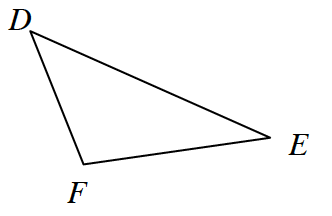### Home > INT1 > Chapter 7 > Lesson 7.1.4 > Problem7-49

7-49.

Examine the triangle at right. Each part is a separate problem.

Review the Math Notes Box in this Lesson 7.1.4.

1. If $m∠D = 48º$ and $m∠F = 117º$, then what is $m∠E$?

Use the Triangle Angle Sum Theorem: $48º + 117º + m∠E = 180º$

2. Solve for $x$ if $m∠D = 4x + 2º$, $m∠F = 7x – 8º$, and $m∠E = 4x + 6º$. Then calculate $m∠D$.

Use the same method as part (a).

3. If $m∠D = m∠F = m∠E$, what type of triangle is $ΔFED$?

If all of the angles have equal measure, then all of the sides have equal measure.